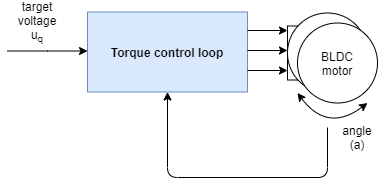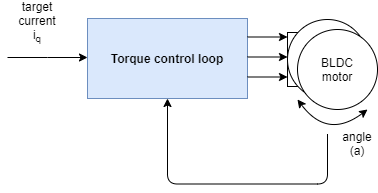# Torque control loop

SimpleFOClibrary gives you the choice of using 3 different torque control strategies:

In short voltage control mode is the simplest approximation of the motor torque control and it so basic that can be run on any motor+driver+mcu combination out there. DC current mode is teh next step of the motor’s torque approximation which is much more exact than the voltage mode but requires current sensing and much stronger microcontroller. FOC current mode is controls motor’s true torque and it is not an approximation, it also requires current sensor and even more processing power than the DC current mode. See in depth explanations in torque mode docs.

This motion control mode is enabled setting the `controller` parameter to:

``````// torque control loop
motor.controller = MotionControlType::torque;
``````

If the voltage control mode is used and if the user does not provide the phase resistance parameter to the motor, the input to the torque control loop will be the target voltage Uq:And if one of the current based torque control modes (DC current or FOC current) is used, the input in the control loop will be the target current iq. The same is true in the voltage mode if the user provides the phase resistance value to the motor class.The torque control loop is used as a base for all other motion control loops. For more info about the content of the blue boxes check the torque mode docs.

## Configuration parameters

Depending on the torque control type you wish to use there are different parameters that you need to consider.

Now, lets see one example!

## Voltage control example

You can test this algorithm by running the example `voltage_control.ino`.

Here we provide an example of a toque using voltage control program with full motion control configuration. The program sets target Uq voltage of 2V to the motor using the FOC algorithm. Since the phase resistance parameter is not available the motor target will be in volts.

``````#include <SimpleFOC.h>

// BLDC motor & driver instance
BLDCMotor motor = BLDCMotor(11);
BLDCDriver3PWM driver = BLDCDriver3PWM(9, 5, 6, 8);

// encoder instance
Encoder encoder = Encoder(2, 3, 500);
// channel A and B callbacks
void doA(){encoder.handleA();}
void doB(){encoder.handleB();}

void setup() {

// initialize encoder sensor hardware
encoder.init();
encoder.enableInterrupts(doA, doB);
// link the motor to the sensor

// driver config
// power supply voltage [V]
driver.voltage_power_supply = 12;
driver.init();

// set the torque control type
motor.torque_controller = TorqueControlType::voltage;
// set motion control loop to be used
motor.controller = MotionControlType::torque;

// use monitoring with serial
Serial.begin(115200);
// comment out if not needed
motor.useMonitoring(Serial);

// initialize motor
motor.init();
// align sensor and start FOC
motor.initFOC();

Serial.println(F("Target voltage is 2V"));
_delay(1000);
}

void loop() {

// main FOC algorithm function
motor.loopFOC();

// Motion control function
motor.move(2);
}
``````

If we add the pahse resitance to the constructor of the `BLDCMotor` class, the motor target will be in Amps.

``````#include <SimpleFOC.h>

// BLDC motor & driver instance
BLDCMotor motor = BLDCMotor(11, 12.34); // 12.34 Ohms
BLDCDriver3PWM driver = BLDCDriver3PWM(9, 5, 6, 8);

// encoder instance
Encoder encoder = Encoder(2, 3, 500);
// channel A and B callbacks
void doA(){encoder.handleA();}
void doB(){encoder.handleB();}

void setup() {

// initialize encoder sensor hardware
encoder.init();
encoder.enableInterrupts(doA, doB);
// link the motor to the sensor

// driver config
// power supply voltage [V]
driver.voltage_power_supply = 12;
driver.init();

// set the torque control type
motor.torque_controller = TorqueControlType::voltage;
// set motion control loop to be used
motor.controller = MotionControlType::torque;

// use monitoring with serial
Serial.begin(115200);
// comment out if not needed
motor.useMonitoring(Serial);

// initialize motor
motor.init();
// align sensor and start FOC
motor.initFOC();

Serial.println(F("Target current is 0.5Amps"));
_delay(1000);
}

void loop() {

// main FOC algorithm function
motor.loopFOC();

// Motion control function
motor.move(0.5);
}
``````

## Project examples

Here is one very cool project example which uses torque control and describes the full hardware + software setup needed.

Find more projects in the example projects section.